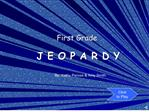# J E O P A R D Y - PowerPoint PPT PresentationDownload PresentationJ E O P A R D Y

J E O P A R D Y
Download Presentation## J E O P A R D Y

- - - - - - - - - - - - - - - - - - - - - - - - - - - E N D - - - - - - - - - - - - - - - - - - - - - - - - - - -
##### Presentation Transcript

1. J E O P A R D Y First Grade

4. Which comes next in the pattern? Patterns - 100

6. Patterns - 100

7. Which comes next in the pattern, basketballs or soccer balls? Patterns - 200

9. Patterns - 200

10. What comes next in the pattern? Patterns - 300

12. Patterns - 300

13. 5 + 3 = ? Math Facts � 100

15. Math Facts � 100

16. 4 + 2 = ? Math Facts � 200

18. Math Facts � 200

19. 10 + 10 = ? Math Facts � 300

21. Math Facts � 300

22. There are 6 stars. There are 8 hearts. How many more hearts than stars? Word Problems � 100

24. Word Problems � 100

25. There are 8 bunnies. Half are running. How many are not running? Word Problems� 200

27. Word Problems � 200

28. You have 2 dimes and 2 nickels. How much money do you have? Word Problems� 300

30. Word Problems � 300

31. A monkey ate 3 bananas at 3 o�clock. An hour later he ate 5 more. What time did he eat the 5 bananas? Bonus Question - 500

33. Bonus Question - 500